# 3.6E: Exercises

•• OpenStax
• OpenStax
$$\newcommand{\vecs}{\overset { \rightharpoonup} {\mathbf{#1}} }$$ $$\newcommand{\vecd}{\overset{-\!-\!\rightharpoonup}{\vphantom{a}\smash {#1}}}$$$$\newcommand{\id}{\mathrm{id}}$$ $$\newcommand{\Span}{\mathrm{span}}$$ $$\newcommand{\kernel}{\mathrm{null}\,}$$ $$\newcommand{\range}{\mathrm{range}\,}$$ $$\newcommand{\RealPart}{\mathrm{Re}}$$ $$\newcommand{\ImaginaryPart}{\mathrm{Im}}$$ $$\newcommand{\Argument}{\mathrm{Arg}}$$ $$\newcommand{\norm}{\| #1 \|}$$ $$\newcommand{\inner}{\langle #1, #2 \rangle}$$ $$\newcommand{\Span}{\mathrm{span}}$$ $$\newcommand{\id}{\mathrm{id}}$$ $$\newcommand{\Span}{\mathrm{span}}$$ $$\newcommand{\kernel}{\mathrm{null}\,}$$ $$\newcommand{\range}{\mathrm{range}\,}$$ $$\newcommand{\RealPart}{\mathrm{Re}}$$ $$\newcommand{\ImaginaryPart}{\mathrm{Im}}$$ $$\newcommand{\Argument}{\mathrm{Arg}}$$ $$\newcommand{\norm}{\| #1 \|}$$ $$\newcommand{\inner}{\langle #1, #2 \rangle}$$ $$\newcommand{\Span}{\mathrm{span}}$$$$\newcommand{\AA}{\unicode[.8,0]{x212B}}$$

## Practice Makes Perfect

Use the Vertical Line Test

In the following exercises, determine whether each graph is the graph of a function.

1. ⓐⓐ no ⓑ yes

2. ⓐ3. ⓐⓐ no ⓑ yes

4. ⓐ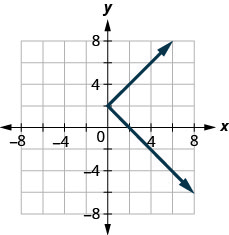Identify Graphs of Basic Functions

In the following exercises, ⓐ graph each function ⓑ state its domain and range. Write the domain and range in interval notation.

5. $$f(x)=3x+4$$ⓑ $$D:(-\inf ,\inf ),\space R:(-\inf ,\inf )$$

6. $$f(x)=2x+5$$

7. $$f(x)=−x−2$$ⓑ $$D:(-\inf ,\inf ),\space R:(-\inf ,\inf )$$

8. $$f(x)=−4x−3$$

9. $$f(x)=−2x+2$$ⓑ $$D:(-\inf ,\inf ),\space R:(-\inf ,\inf )$$

10. $$f(x)=−3x+3$$

11. $$f(x)=\frac{1}{2}x+1$$ⓑ $$D:(-\inf ,\inf ),\space R:(-\inf ,\inf )$$

12. $$f(x)=\frac{2}{3}x−2$$

13. $$f(x)=5$$ⓑ $$D:(-\inf ,\inf ), R:{5}$$

14. $$f(x)=2$$

15. $$f(x)=−3$$ⓑ $$D:(-\inf ,\inf ),\space R: {−3}$$

16. $$f(x)=−1$$

17. $$f(x)=2x$$ⓑ $$D:(-\inf ,\inf ),\space R:(-\inf ,\inf )$$

18. $$f(x)=3x$$

19. $$f(x)=−2x$$ⓑ $$D:(-\inf ,\inf ), R:(-\inf ,\inf )$$

20. $$f(x)=−3x$$

21. $$f(x)=3x^2$$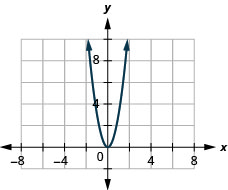ⓑ $$D:(-\inf ,\inf ),\space R:[0,\inf )$$

22. $$f(x)=2x^2$$

23. $$f(x)=−3x^2$$ⓑ $$D: (-\inf ,\inf ),\space R:(-\inf ,0]$$

24. $$f(x)=−2x^2$$

25. $$f(x)=12x^2$$ⓑ $$D: (-\inf ,\inf ),\space R:[-\inf ,0)$$

26. $$f(x)=\frac{1}{3}x^2$$

27. $$f(x)=x^2−1$$ⓑ $$D: (-\inf ,\inf ),\space R:[−1, \inf )$$

28. $$f(x)=x^2+1$$

29. $$f(x)=−2x^3$$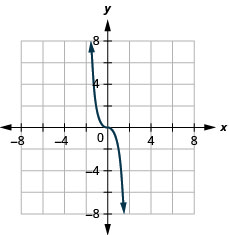ⓑ $$D:(-\inf ,\inf ),\space R:(-\inf ,\inf )$$

30. $$f(x)=2x^3$$

31. $$f(x)=x^3+2$$ⓑ $$D:(-\inf ,\inf ), R:(-\inf ,\inf )$$

32. $$f(x)=x^3−2$$

33. $$f(x)=2\sqrt{x}$$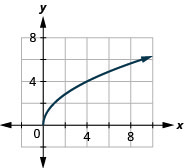ⓑ $$D:[0,\inf ), R:[0,\inf )$$

34. $$f(x)=−2\sqrt{x}$$

35. $$f(x)=\sqrt{x-1}$$ⓑ $$D:[1,\inf ), R:[0,\inf )$$

36. $$f(x)=\sqrt{x+1}$$

37. $$f(x)=3|x|$$ⓑ $$D:[ −1,−1, \inf ), R:[−\inf ,\inf )$$

38. $$f(x)=−2|x|$$

39. $$f(x)=|x|+1$$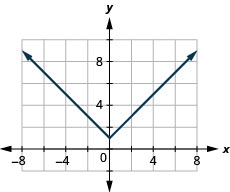ⓑ $$D:(-\inf ,\inf ), R:[1,\inf )$$

40. $$f(x)=|x|−1$$

Read Information from a Graph of a Function

In the following exercises, use the graph of the function to find its domain and range. Write the domain and range in interval notation.

41.$$D: [2,\inf ),\space R: [0,\inf )$$

42.43.$$D: (-\inf ,\inf ),\space R: [4,\inf )$$

44.45.$$D: [−2,2],\space R: [0, 2]$$

46.In the following exercises, use the graph of the function to find the indicated values.

47.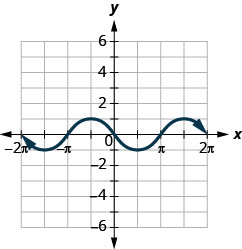ⓐ Find: $$f(0)$$.
ⓑ Find: $$f(12\pi)$$.
ⓒ Find: $$f(−32\pi)$$.
ⓓ Find the values for $$x$$ when $$f(x)=0$$.
ⓔ Find the $$x$$-intercepts.
ⓕ Find the $$y$$-intercepts.
ⓖ Find the domain. Write it in interval notation.
ⓗ Find the range. Write it in interval notation.

ⓐ $$f(0)=0$$ ⓑ $$(\pi/2)=−1$$
ⓒ $$f(−3\pi/2)=−1$$ ⓓ $$f(x)=0$$ for $$x=−2\pi,-\pi,0,\pi,2\pi$$
ⓔ $$(−2\pi,0),(−\pi,0),$$ $$(0,0),(\pi,0),(2\pi,0)$$ $$(f)(0,0)$$
ⓖ $$[−2\pi,2\pi]$$ ⓗ $$[−1,1]$$

48.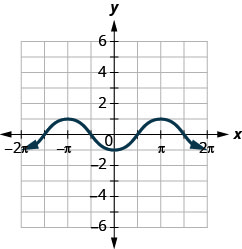ⓐ Find: $$f(0)$$.
ⓑ Find: $$f(\pi)$$.
ⓒ Find: $$f(−\pi)$$.
ⓓ Find the values for $$x$$ when $$f(x)=0$$.
ⓔ Find the $$x$$-intercepts.
ⓕ Find the $$y$$-intercepts.
ⓖ Find the domain. Write it in interval notation.
ⓗ Find the range. Write it in interval notation

49.ⓐ Find: $$f(0)$$.
ⓑ Find: $$f(−3)$$.
ⓒ Find: $$f(3)$$.
ⓓ Find the values for $$x$$ when $$f(x)=0$$.
ⓔ Find the $$x$$-intercepts.
ⓕ Find the $$y$$-intercepts.
ⓖ Find the domain. Write it in interval notation.
ⓗ Find the range. Write it in interval notation.

ⓐ $$f(0)=−6$$ ⓑ $$f(−3)=3$$ ⓒ $$f(3)=3$$ ⓓ $$f(x)=0$$ for no x ⓔ none ⓕ $$y=6$$ ⓖ $$[−3,3]$$
ⓗ $$[−3,6]$$

50.ⓐ Find: $$f(0)$$.
ⓑ Find the values for $$x$$ when $$f(x)=0$$.
ⓒ Find the $$x$$-intercepts.
ⓓ Find the $$y$$-intercepts.
ⓔ Find the domain. Write it in interval notation.
ⓕ Find the range. Write it in interval notation

## Writing Exercises

51. Explain in your own words how to find the domain from a graph.

52. Explain in your own words how to find the range from a graph.

53. Explain in your own words how to use the vertical line test.

54. Draw a sketch of the square and cube functions. What are the similarities and differences in the graphs?

## Self Check

ⓐ After completing the exercises, use this checklist to evaluate your mastery of the objectives of this section.ⓑ After reviewing this checklist, what will you do to become confident for all objectives?

This page titled 3.6E: Exercises is shared under a CC BY license and was authored, remixed, and/or curated by OpenStax.